# 送机器学习电子书——(TensorFlow)RNN入门

+关注继续查看1.上下文信息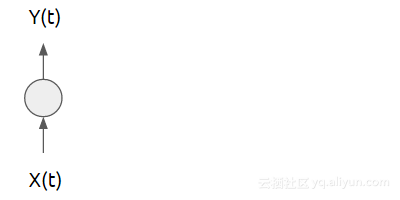1分别具有标记为Xk）和Yk）的输入和输出层的神经网络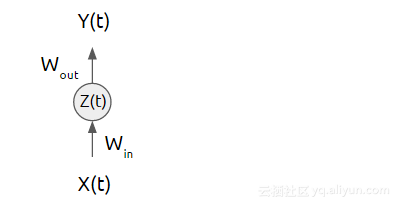2神经网络的隐藏层可以被认为是数据的隐藏，由其输入权重编码并输出权重解码。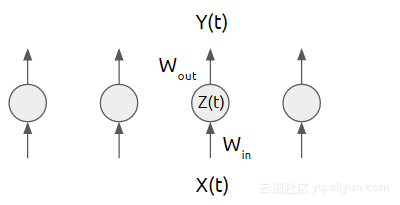3通常，我们会运行相同的神经网络多次，而不考虑关于先前运行的隐藏状态。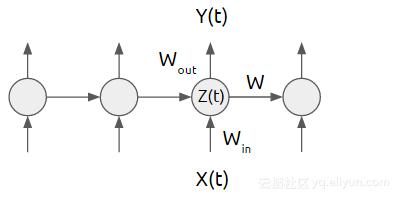4循环神经网络架构可以利用网络的先前状态来实现其优点。

2.实施循环神经网络

RNN的一种类型模型被称为长短期记忆网络（LSTM）。我觉得这是一个有趣的名字。它听起来也意味着：短期模式长期不会被遗忘。

LSTM的精确实现细节不在本文的范围之内。相信我，如果只学习LSTM模型会分散我们的注意力，因为它还没有确定的标准。

import numpy as np
import tensorflow as tf
from tensorflow.contrib import rnn


class SeriesPredictor:
def __init__(self, input_dim, seq_size, hidden_dim=10):
self.input_dim = input_dim //#A
self.seq_size = seq_size  //#A
self.hidden_dim = hidden_dim  //#A
self.W_out = tf.Variable(tf.random_normal([hidden_dim, 1]),name='W_out') //#B
self.b_out = tf.Variable(tf.random_normal(), name='b_out')  //#B
self.x = tf.placeholder(tf.float32, [None, seq_size, input_dim]) //#B
self.y = tf.placeholder(tf.float32, [None, seq_size]) //#B
self.cost = tf.reduce_mean(tf.square(self.model() - self.y)) //#C
self.saver = tf.train.Saver()  //#D


#A超参数。

#B权重变量和输入占位符。

#C成本优化器（cost optimizer）。

#D辅助操作。

def model(self):
"""
:param x: inputs of size [T, batch_size, input_size]
:param W: matrix of fully-connected output layer weights
:param b: vector of fully-connected output layer biases
"""
cell = rnn.BasicLSTMCell(self.hidden_dim)  #A
outputs, states = tf.nn.dynamic_rnn(cell, self.x, dtype=tf.float32) #B
num_examples = tf.shape(self.x)
W_repeated = tf.tile(tf.expand_dims(self.W_out, 0), [num_examples, 1, 1])#C
out = tf.matmul(outputs, W_repeated) + self.b_out
out = tf.squeeze(out)
return out


#A创建一个LSTM单元。

#B运行输入单元，获取输出和状态的张量。

#C将输出层计算为完全连接的线性函数。

def train(self, train_x, train_y):
with tf.Session() as sess:
tf.get_variable_scope().reuse_variables()
sess.run(tf.global_variables_initializer())
for i in range(1000):  #A
mse = sess.run([self.train_op, self.cost], feed_dict={self.x: train_x, self.y: train_y})
if i % 100 == 0:
print(i, mse)
save_path = self.saver.save(sess, 'model.ckpt')
print('Model saved to {}'.format(save_path))

#A训练1000

def test(self, test_x):
with tf.Session() as sess:
tf.get_variable_scope().reuse_variables()
self.saver.restore(sess, './model.ckpt')
output = sess.run(self.model(), feed_dict={self.x: test_x})
print(output)


if __name__ == '__main__':
predictor = SeriesPredictor(input_dim=1, seq_size=4, hidden_dim=10)
train_x = [[, , , ],
[, , , ],
[, , , ]]
train_y = [[1, 3, 7, 11],
[5, 12, 14, 15],
[3, 7, 9, 12]]
predictor.train(train_x, train_y)
test_x = [[, , , ],  #A
[, , , ]]  #B
predictor.test(test_x)


#A预测结果应为1357

#B预测结果应为491113

Machine Learning with TensorFlow

## 文章原标题：《Recurrent Neural Networks》35 0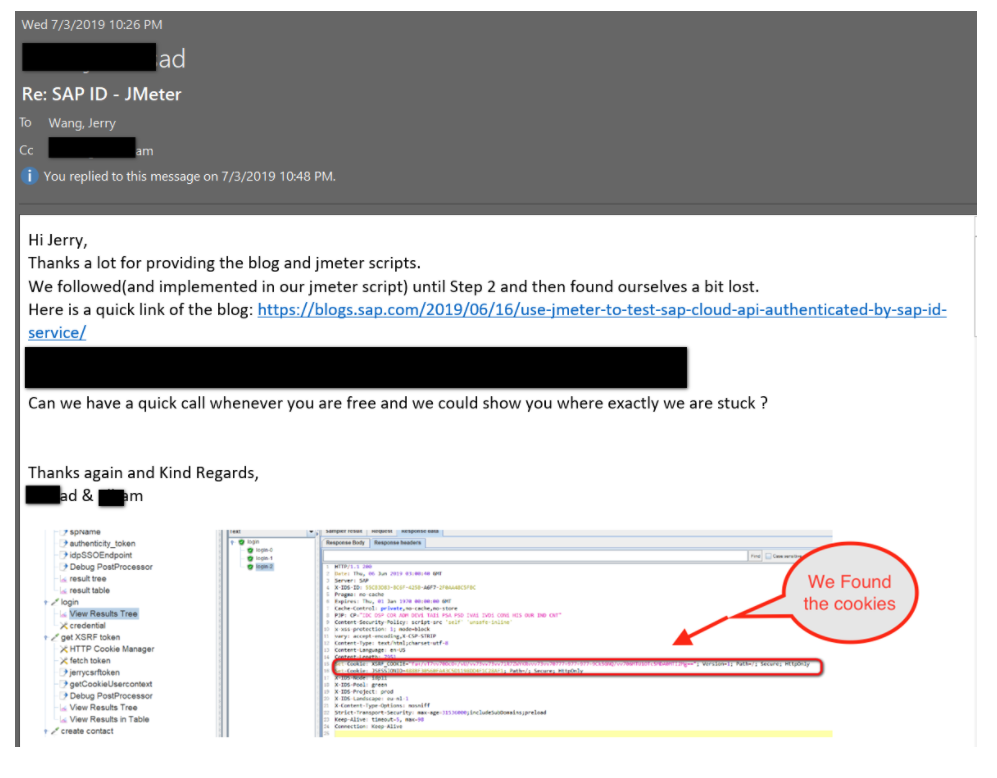3 0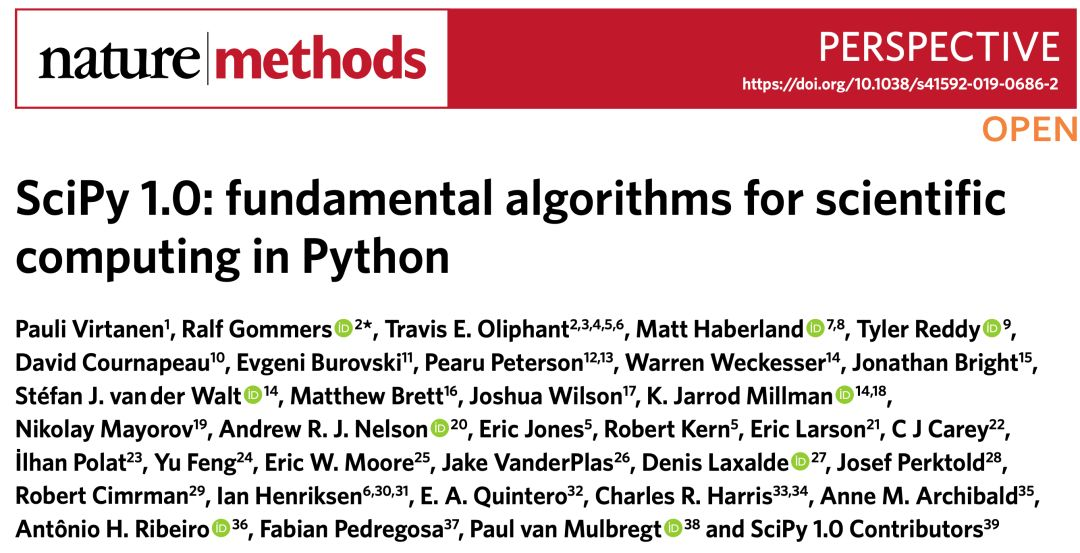7 0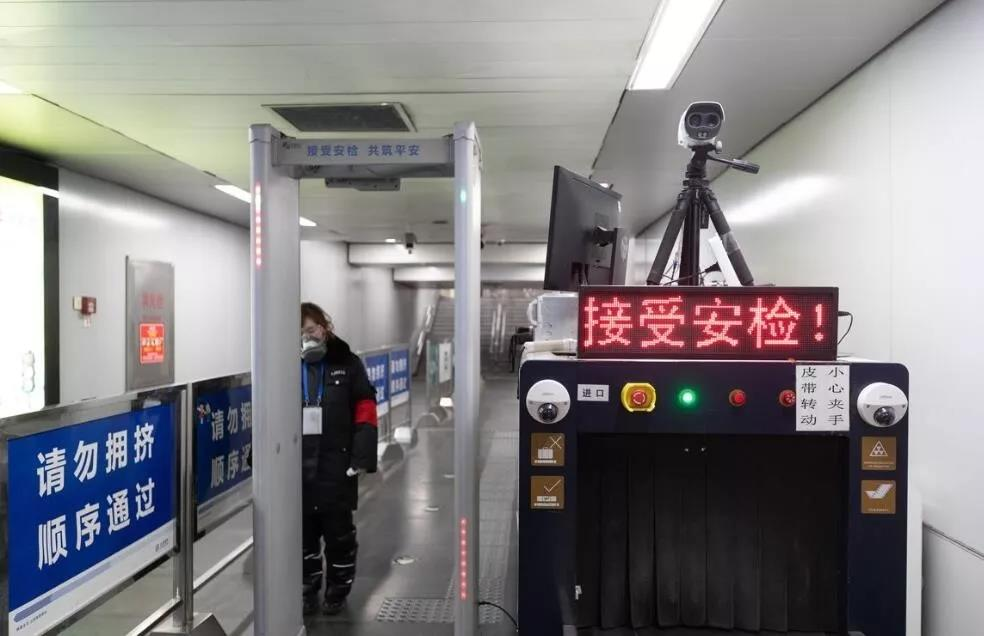2月10日返工潮即将到来，北京开始部署「快速AI体温检测仪」
AI 技术正在帮助大量即将返回工作岗位的人们，让接触新冠病毒的风险不断降低。
4 03 03 0
【方向】

696

5

《SaaS模式云原生数据仓库应用场景实践》

《看见新力量：二》电子书